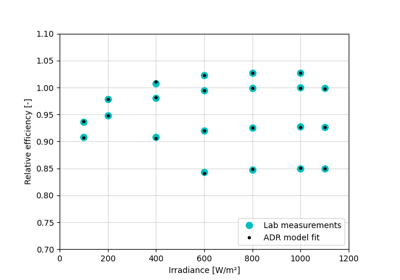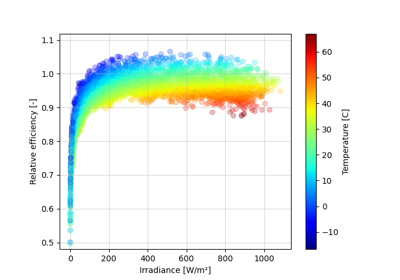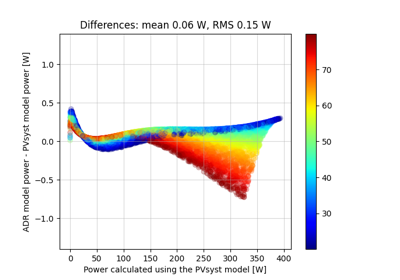Calculate PV module efficiency using the ADR model.

The efficiency varies with irradiance and operating temperature and is determined by 5 model parameters as described in 1.

Parameters
• effective_irradiance (numeric, non-negative) – The effective irradiance incident on the PV module. [W/m^2]

• temp_cell (numeric) – The PV module operating temperature. [°C]

• k_a (numeric) – Absolute scaling factor, which is equal to the efficiency at reference conditions. This factor allows the model to be used with relative or absolute efficiencies, and to accommodate data sets which are not perfectly normalized but have a slight bias at the reference conditions. [unitless]

• k_d (numeric, negative) – “Dark irradiance” or diode coefficient which influences the voltage increase with irradiance. [unitless]

• tc_d (numeric) – Temperature coefficient of the diode coefficient, which indirectly influences voltage. Because it is the only temperature coefficient in the model, its value will also reflect secondary temperature dependencies that are present in the PV module. [unitless]

• k_rs (numeric) – Series resistance loss coefficient. Because of the normalization it can be read as a power loss fraction at reference conditions. For example, if `k_rs` is 0.05, the internal loss assigned to the series resistance has a magnitude equal to 5% of the module output. [unitless]

• k_rsh (numeric) – Shunt resistance loss coefficient. Can be interpreted as a power loss fraction at reference conditions like `k_rs`. [unitless]

Returns

eta (numeric) – The efficiency of the module at the specified irradiance and temperature.

Notes

Efficiency values `eta` may be absolute or relative, and may be expressed as percent or per unit. This is determined by the efficiency data used to derive values for the 5 model parameters. The first model parameter `k_a` is equal to the efficiency at STC and therefore indicates the efficiency scale being used. `k_a` can also be changed freely to adjust the scale, or to change the module to a slightly higher or lower efficiency class.

All arguments may be scalars or array-like. If multiple arguments are array-like they must be the same shape or broadcastable to the same shape.

References

1

A. Driesse and J. S. Stein, “From IEC 61853 power measurements to PV system simulations”, Sandia Report No. SAND2020-3877, 2020. DOI: 10.2172/1615179

2

A. Driesse, M. Theristis and J. S. Stein, “A New Photovoltaic Module Efficiency Model for Energy Prediction and Rating,” in IEEE Journal of Photovoltaics, vol. 11, no. 2, pp. 527-534, March 2021. DOI: 10.1109/JPHOTOV.2020.3045677

Examples

```>>> pvefficiency_adr([1000, 200], 25,
k_a=100, k_d=-6.0, tc_d=0.02, k_rs=0.05, k_rsh=0.10)
array([100.        ,  92.79729308])
```
```>>> pvefficiency_adr([1000, 200], 25,
k_a=1.0, k_d=-6.0, tc_d=0.02, k_rs=0.05, k_rsh=0.10)
array([1.        , 0.92797293])
```

## Examples using `pvlib.pvarray.pvefficiency_adr`#Obtaining ADR model parameters from IEC 61853 matrix measurements

Obtaining ADR model parameters from IEC 61853 matrix measurementsSimulating PV system DC output using the ADR module efficiency model

Simulating PV system DC output using the ADR module efficiency modelFast simulation using the ADR efficiency model starting from PVsyst parameters

Fast simulation using the ADR efficiency model starting from PVsyst parameters